Algebra 1 5-8 Complete Lesson: Graphing Absolute Value Functions
starstarstarstarstarstarstarstarstarstar
4.5 (1 rating)
by Matthew Richardson
| 27 Questions
Note from the author:
A complete formative lesson with embedded slideshow, mini lecture screencasts, checks for understanding, practice items, mixed review, and reflection. I create these assignments to supplement each lesson of Pearson's Common Core Edition Algebra 1, Algebra 2, and Geometry courses. See also mathquest.net and twitter.com/mathquestEDU.The outlined content above was added from outside of Formative.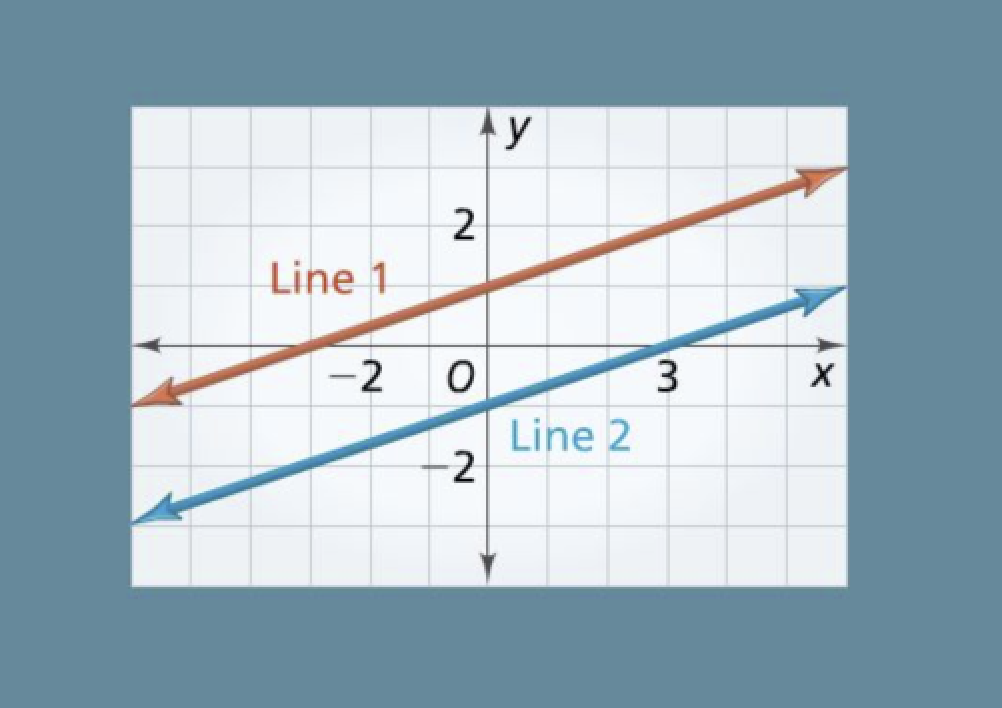1
2
3
4
1
10 pts
Solve It! Write the equation of Line 1 in slope-intercept form.
y = ½x + 1
y = ⅓x + 1
y = 3x + 2
y = 2x + 1
2
10 pts
Solve It! Write the equation of Line 2 in slope-intercept form.
3
10 pts
Solve It! How can you transform the equation of Line 1 into the equation of Line 2? Explain.
4
10 pts
Solve It! How can you slide Line 1 in the coordinate plane so that it becomes Line 2? Explain.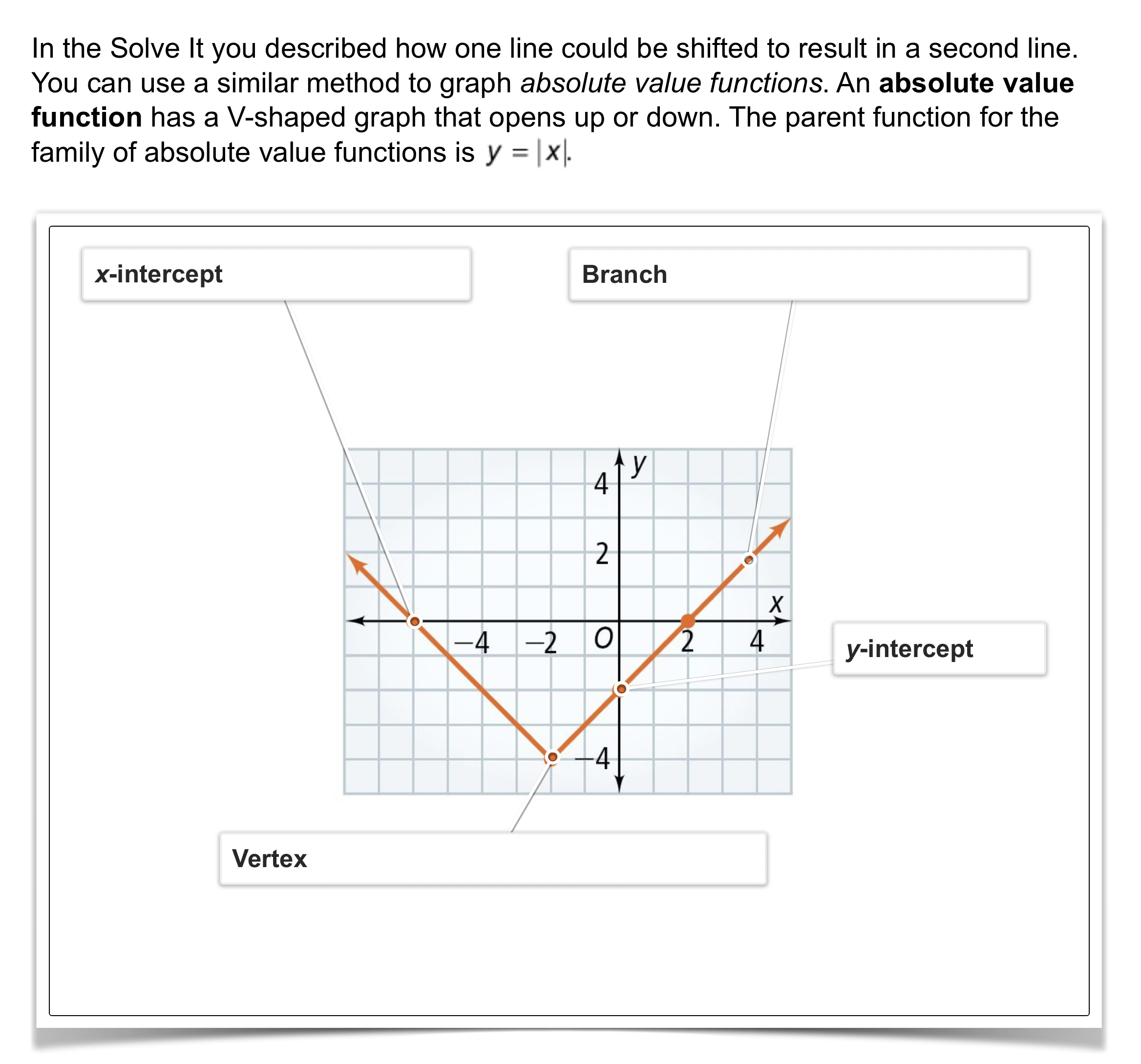5
10 pts
Vocabulary: In your own words, define translation.
6
10 pts
Graphing: Graph the absolute value function. Zoom and pan your graph to establish an appropriate viewing window.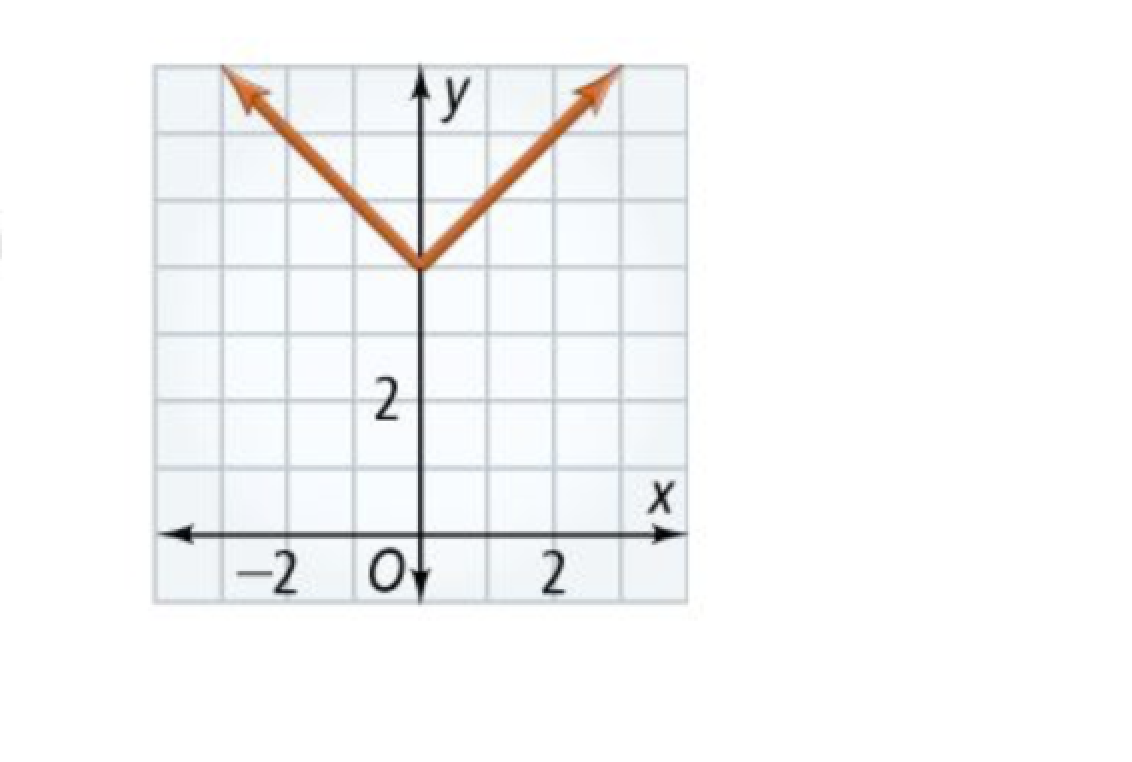7
7
10 pts
Problem 1 Got It? How is the graph shown related to the graph of y = |x|?
The graph shown is the graph of y = |x| shifted right 4 units.
The graph shown is the graph of y = |x| shifted down 4 units.
The graph shown is the graph of y = |x| shifted up 4 units.
The graph shown is the graph of y = |x| shifted left 4 units.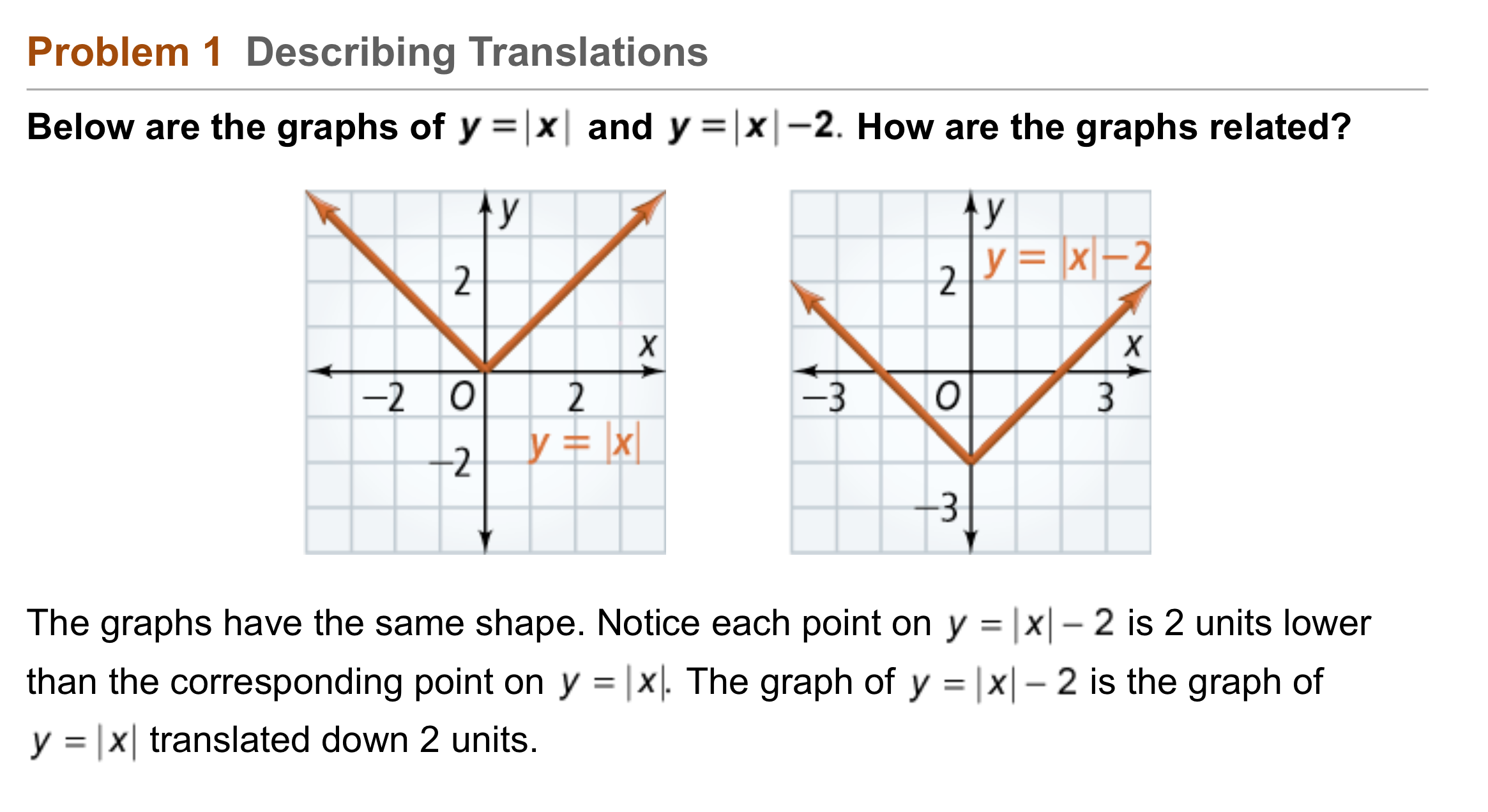8
8
10 pts
Problem 1 Got It? Reasoning: Match the domains and ranges on the left with the appropriate function(s) on the right from Problem 1. Not all domains and ranges will be used.
• D: All real numbers
• R: All real numbers
• D: [0, ∞)
• R: [0, ∞)
• R: [-2, ∞)
• y = |x|
• y = |x| - 2
9
10 pts
Problem 2 Got It?
Be sure to include relevant graph detail: label axes, indicate units on both axes, and use arrows to represent end behavior, as appropriate.10
10 pts
Problem 3 Got It?
Be sure to include relevant graph detail: label axes, indicate units on both axes, and use arrows to represent end behavior, as appropriate.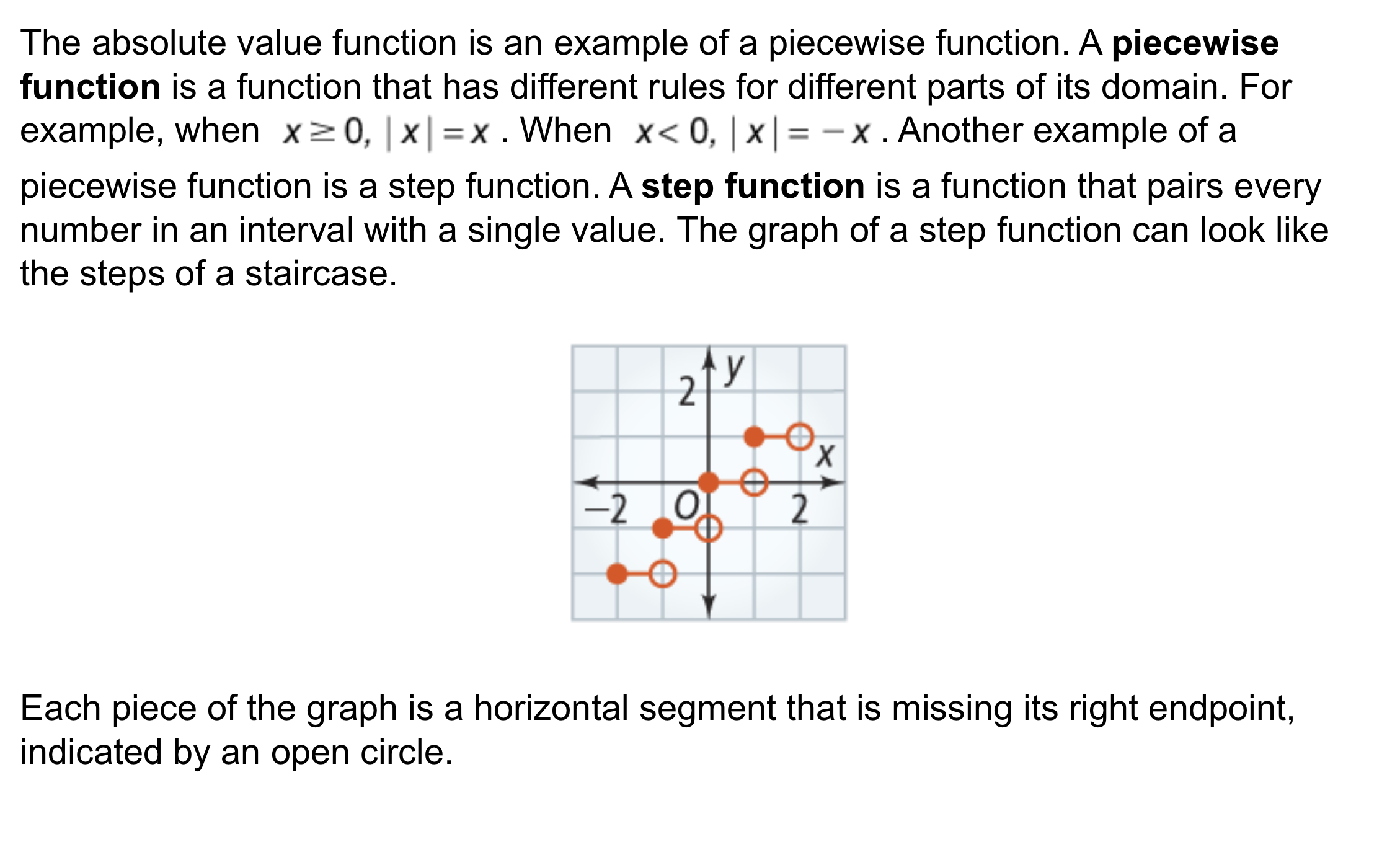11
10 pts
Problem 4 Got It? Make a graph that models the relationship between the number of students x that go to the game by bus and the number of busses y that are needed if each bus holds a maximum of 50 students.

Be sure to include relevant graph detail: label axes, indicate units on both axes, and use arrows to represent end behavior, as appropriate.12
10 pts
How is the graph of y = |x| - 8 different from the graph of y = |x|?
How is it the same?

After responding, you may check your answer using Desmos below. If you must edit your response, consider also editing your note card.
The outlined content above was added from outside of Formative.
13
10 pts
What is the equation for the translation of y = |x| 9 units up?
You may check your response using Desmos.
y = |x + 9|
y = |x| - 9
y = |x| + 9
y = |x - 9|
14
10 pts
What is the graph of the function?
Be sure to include relevant graph detail: label axes, indicate units on both axes, and use arrows to represent end behavior, as appropriate.15
10 pts
Compare and Contrast: How are the graphs of y = |x| - 4 and y = |x - 4| the same? How are they different?
16
10 pts
Error Analysis: A student is graphing the equation y = |x - 10| and translates the graph of y = |x| 10 units left. Describe her error.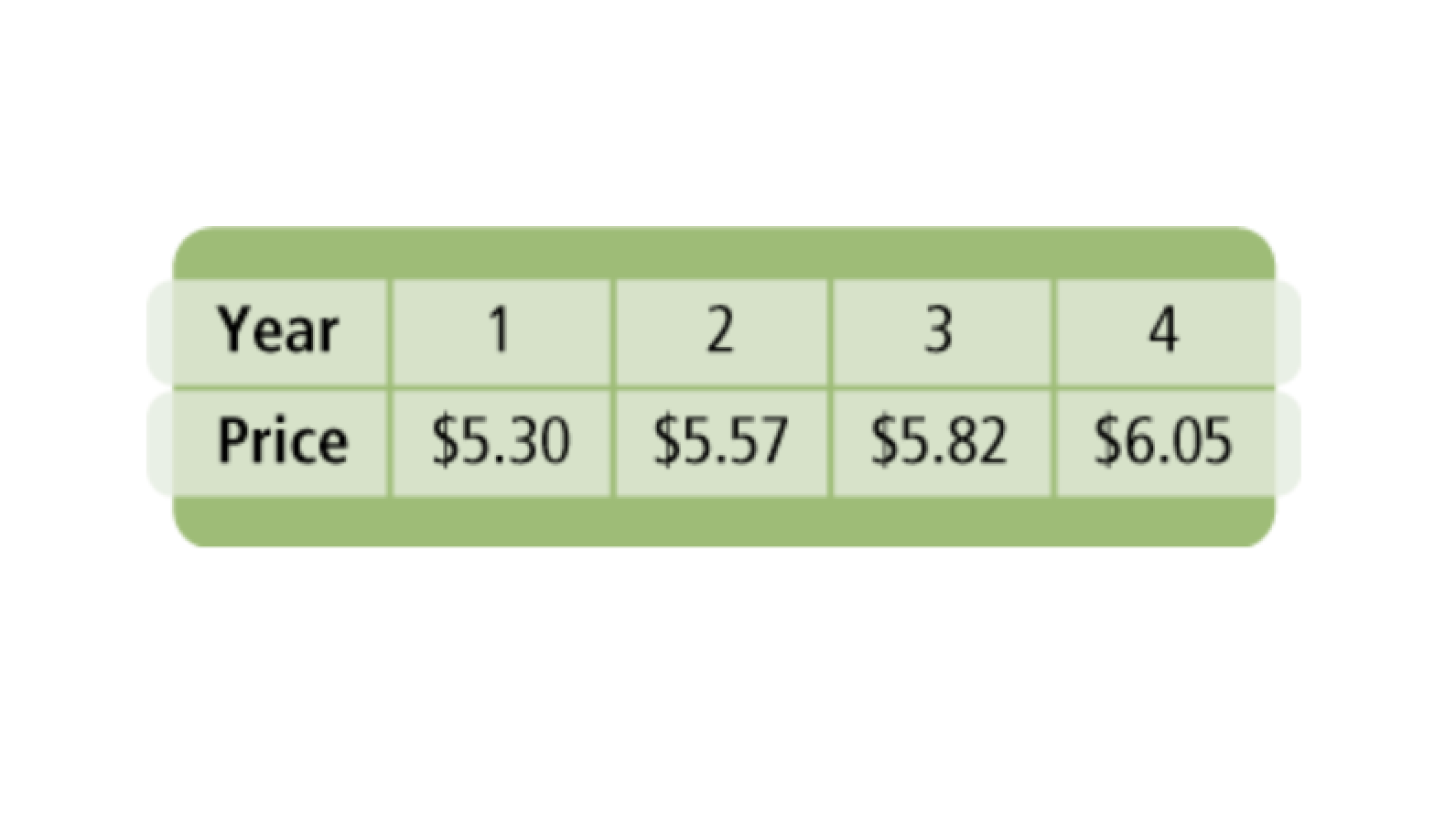17
17
10 pts
Reveiw Lesson 5-7: Write an equation of a trend line or use a graphing calculator to find an equation of the line of best fit.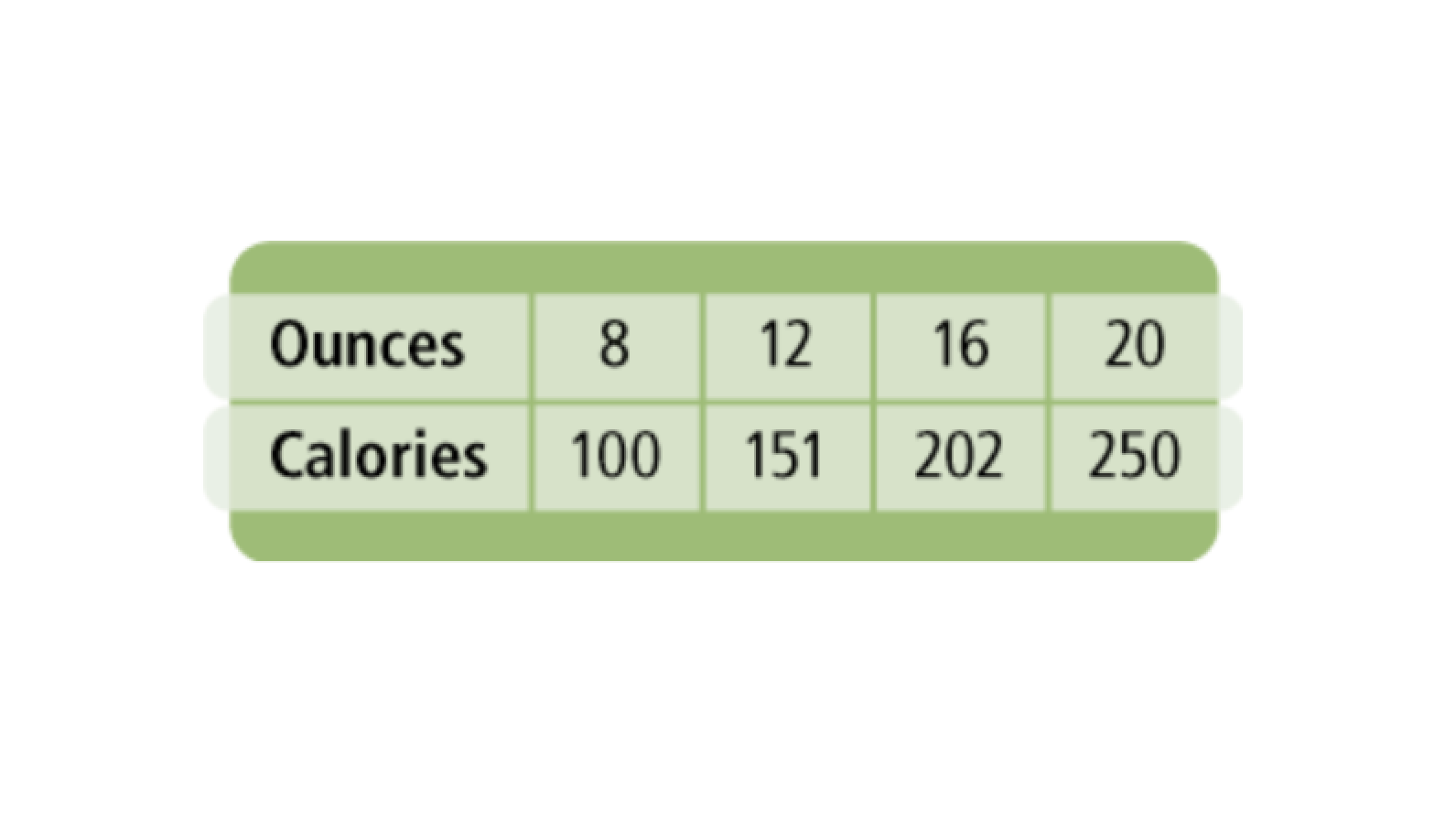18
18
10 pts
Reveiw Lesson 5-7: Write an equation of a trend line or use a graphing calculator to find an equation of the line of best fit.
19
40 pts
Review Lesson 5-3: Graph the equations on the same coordinate plane using the colors indicated for each.

Be sure to include relevant graph detail: label axes, indicate units on both axes, and use arrows to represent end behavior, as appropriate.20
10 pts
Vocabulary Review: Compare the absolute values using the symbols on the left.
• <
• >
• =
• |3| _?_ |-3|
• |-3| _?_ |-1|
• |9| _?_ |-10|
• |-9| _?_ |8|
21
5 pts
Vocabulary Review: The absolute value of a number is a measure of the distance from 0 to that number on the number line.
True
False
22
5 pts
Vocabulary Review: The absolute value of a number is always the opposite of that number.
True
False23
24
25
23
5 pts
Use Your Vocabulary: Classify the pair of figures.
translation
not a translation
24
5 pts
Use Your Vocabulary: Classify the pair of figures.
translation
not a translation
25
5 pts
Use Your Vocabulary: Classify the pair of figures.
translation
not a translation
26
100 pts
Notes: Take a clear picture or screenshot of your Cornell notes for this lesson. Upload it to the canvas. Zoom and pan as needed.

For a refresher on the Cornell note-taking system, click here.
27
10 pts
Reflection: Math Success
Add to my formatives list

Formative uses cookies to allow us to better understand how the site is used. By continuing to use this site, you consent to the Terms of Service and Privacy Policy.